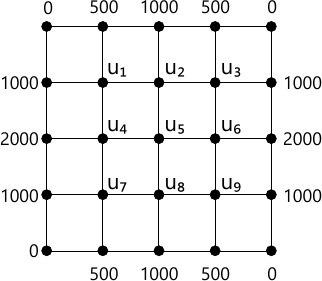MORE IN Engineering Mathematics 3
VTU Computer Science (Semester 3)
Engineering Mathematics 3
December 2015
Total marks: --
Total time: --
INSTRUCTIONS
(1) Assume appropriate data and state your reasons
(2) Marks are given to the right of every question
(3) Draw neat diagrams wherever necessary

1 (a) For the function: $f(x)= \left\{\begin{matrix} x \ \ \ in &0< x< \pi \\ x-2\pi n & \pi < x< 2\pi \end{matrix}\right.$ Find the Fourier series expansion and hence deduce the result $$\dfrac {\pi}{4}= 1 - \dfrac {1}{3} + \dfrac {1}{5} - \cdots \ \cdots$$
7 M
1 (b) Obtain the half range Fourier cosine series of the function f(x)=x(l-x) in 0 ≤ x ≤ l.
6 M
1 (c) Find the constant term and first harmonic term in the Fourier expansion of y from the following table:
 x 0 1 2 3 4 5 y 9 18 24 28 26 20
7 M

2 (a) Find the Fourier transform of the function: $$\left\{\begin{matrix} 1 \text{ for}&|x|\lt a \\ 0 \text{ for}&|x| \gt a \end{matrix}\right.$$ and hence evaluate: $$\int^{\infty}_0 \dfrac {\sin x}{x}dx$$
7 M
2 (b) Obtain the Fourier sine transform of f(x)=e-|x| and hence evaluate $\int^{\infty}_0 \dfrac {x \sin mx}{1+x^2} dx, \ m>0$
7 M
2 (c) Solve the integral equation: $\int^{\infty}_0 f(x) \cos pxdx = \left\{\begin{matrix} 1-p, &0\le p\le l \\0, & p>1 \end{matrix}\right.$ and hence deduce the value $\int^{\infty}_0 \dfrac {\sin^2 t}{t^2}dt.$
7 M

3 (a) Obtain the various possible solutions of the two dimensional Laplace's equation uxx+uyy=0 by the method of separation of variables.
7 M
3 (b) A string is stretched and fastened to two points 'l' apart. Motion is started by displacing the string in the form $$y=a \sin \left ( \dfrac {\pi x}{l} \right )$$ from which it is released at time t=0. Show that the displacement of any point at a distance 'x' from one end at time 't' is given by $$y(x, t)=a \sin \left ( \dfrac {\pi x}{l} \right )\cos \left ( \dfrac {\pi ct}{l} \right ).$$
6 M
3 (c) Obtain the D'Alembert's solution of the wave equation utt=c2 uxx subjected to the conditions u(x, 0)= f(x) and $$u(x, 0) = f(x) \text { and } \dfrac {\partial u}{\partial t}(x, 0)=a.$$
7 M

4 (a) For the following data fit an exponential curve of the form y=a ebx by the method of least squares:
 x 5 6 7 8 9 10 y 133 55 23 7 2 2
7 M
4 (b) Solve the following LPP graphically:
Minimize Z=20x + 10y
Subject to the constraints: x+2y≤40
3x+y≥30
4x+3y≥60
x≥0 and y≥0
6 M
4 (c) Using Simplex method, solve the following LPP:
Maximize: Z=2x+4y
Subject to the constraint
3x+y≤22
2x+3y≤24
x≥0 and y≥0.
7 M

5 (a) Using the Regula-Falsi method to find the fourth root of 12 correct to three decimal places.
7 M
5 (b) Apply Gauss-Seidel method to solve the following of equations correct to three decimal places:
6x+15y+2z=72
x+y+54z=110
27x+6y-z=8.5
(Carry out 3 iterations).
6 M
5 (c) Using Rayleigh power method, determine the largest Eigen value and the corresponding Eigen vector of the matrix A in six iterations. Choose [1 1 1]T as the initial Eigen vector: $A=\begin{bmatrix} 2 &-1 &0 \\-1 &2 &-1 \\0 &-1 &2 \end{bmatrix}$
7 M

6 (a) Using suitable interpolation formulae, find y(38) and y(85) for the following data:
 x 40 50 60 70 80 90 y 184 204 226 250 276 304
7 M
6 (b) If y(0)=-12, y(1)=0, y(3)=6 and y(4)=12, find the Lagrange's interpolation polynomial and estimate y at x=2.
6 M
6 (c) By applying Weddle's rule, evaluate: $$\int^1_0 \dfrac {xdx}{1+x^2}$$ by considering seven ordinates. Hence find the value of loge2.
7 M

7 (a) Using finite difference equation, solve $$\dfrac {\partial^2 u}{\partial t^2 } = 4 \dfrac {\partial ^2 u}{\partial x^2}$$ subject to u(0, t) = u(4, t)=0 ut(x, 0) and u(x, 0) = x(4-x) upto four time steps. Choose h=1 and k=0.5.
7 M
7 (b) Solve the equation ut=uxx subject to the conditions u(0, t)=0, u(1, t)=0, u(x,0)= sin (π x) for 0≤t≤0.1 by taking h=0.2.
6 M
7 (c) Solve the elliptic equation uxx+yyy=0 for the following square mesh with boundary values as shown. Find the first iterative values of u(i=1-9) to the nearest integer.7 M

8 (a) Find the z-transform of 2n+sin (nπ/4)+1.
7 M
8 (b) Obtain the inverse z-transform of $$\dfrac {2z^2 + 3x}{(z+2)(z-4)}$$
6 M
8 (c) Using z-transform, solve the following difference equation:
un+2 + 2un+1 + un=n with n0=u1 =0.
7 M

More question papers from Engineering Mathematics 3